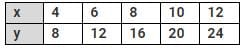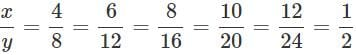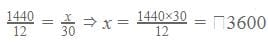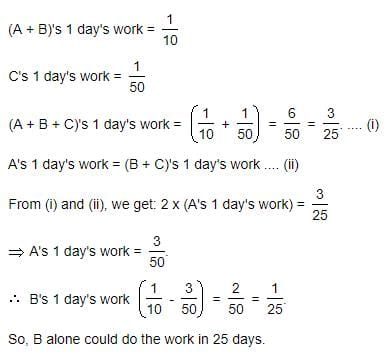Test: Direct And Inverse Proportions- 1

# Test: Direct And Inverse Proportions- 1

Test Description

## 10 Questions MCQ Test Mathematics (Maths) Class 8 | Test: Direct And Inverse Proportions- 1

Test: Direct And Inverse Proportions- 1 for Class 8 2022 is part of Mathematics (Maths) Class 8 preparation. The Test: Direct And Inverse Proportions- 1 questions and answers have been prepared according to the Class 8 exam syllabus.The Test: Direct And Inverse Proportions- 1 MCQs are made for Class 8 2022 Exam. Find important definitions, questions, notes, meanings, examples, exercises, MCQs and online tests for Test: Direct And Inverse Proportions- 1 below.
Solutions of Test: Direct And Inverse Proportions- 1 questions in English are available as part of our Mathematics (Maths) Class 8 for Class 8 & Test: Direct And Inverse Proportions- 1 solutions in Hindi for Mathematics (Maths) Class 8 course. Download more important topics, notes, lectures and mock test series for Class 8 Exam by signing up for free. Attempt Test: Direct And Inverse Proportions- 1 | 10 questions in 10 minutes | Mock test for Class 8 preparation | Free important questions MCQ to study Mathematics (Maths) Class 8 for Class 8 Exam | Download free PDF with solutions
 1 Crore+ students have signed up on EduRev. Have you?
Test: Direct And Inverse Proportions- 1 - Question 1

### If two quantities x and y vary directly, then

Detailed Solution for Test: Direct And Inverse Proportions- 1 - Question 1

If the ratio (x/y) of two variables (x and y) is equal to a constant (k = yx), then the variable in the numerator of the ratio (y) is the product of the other variable and the constant (y = k⋅x). In this case y is said to be directly proportional to x with proportionality constant k.

Test: Direct And Inverse Proportions- 1 - Question 2

### If 8:12::6:a, the value of a is

Test: Direct And Inverse Proportions- 1 - Question 3

### Observe the given table.What can be said about x and y?

Detailed Solution for Test: Direct And Inverse Proportions- 1 - Question 3

Clearly,∴x and y are directly proportional as x/y is a constant.

Test: Direct And Inverse Proportions- 1 - Question 4

If 3 men or 6 women can do a piece of work in 16 days, in how many days can 12 men and 8 women do the same piece of work?

Detailed Solution for Test: Direct And Inverse Proportions- 1 - Question 4

Given, 3 men = 6 women
Now, 12 men = (12 x 6)/3 = 24 women
And 12 men + 8 women = 24 women + 8 women = 32 women.
If, 6 women can do a piece of work in 16 days ---------- (given)
32 women will do that piece of work in = 6 x (16/32) = 3 days
12 men and 8 women will do the piece of work in 3 days.

Test: Direct And Inverse Proportions- 1 - Question 5

A car is traveling at a speed of 54 km/ hour, covers a distance in 2.5 hours. At a speed of 45 km/hr, it covers the same distance in

Test: Direct And Inverse Proportions- 1 - Question 6

12 men can dig 8 meters long trench in a day. How many men should be employed for digging 50 meter long trench of the same type in one day?

Detailed Solution for Test: Direct And Inverse Proportions- 1 - Question 6

This is a question of direct proportion as with increase in depth of digging the number of men will increase
So, in direct proportion the constant value is given by X/ Y
12/8 = a/50 (where a is the number of men to be employed)
12 X 50 = 8 X a
600 = 8 X a
600/ 8 = a
75 men = a

Test: Direct And Inverse Proportions- 1 - Question 7

If the cost of 18 pens is Rs. 234, a dozen pen will cost

Detailed Solution for Test: Direct And Inverse Proportions- 1 - Question 7

Cost of 18 pens = Rs 234
So Cost of 1 pen = 234/18=13
So a dozen pens (12 pens) will cost =
12 X13 = Rs 156

Test: Direct And Inverse Proportions- 1 - Question 8

Raju earns Rs 1440, if he works for 12 days. If he works for 30 days, he will earn

Detailed Solution for Test: Direct And Inverse Proportions- 1 - Question 8

Since wages are directly related to no. of days , we apply direct variationTest: Direct And Inverse Proportions- 1 - Question 9

There is enough food to last for 40 people for 10 days. If 10 more people join them, the food will last for

Test: Direct And Inverse Proportions- 1 - Question 10

A can do a certain work in the same time in which B and C together can do it. If A and B together could do it in 10 days and C alone in 50 days, then B alone could do it in:

Detailed Solution for Test: Direct And Inverse Proportions- 1 - Question 10## Mathematics (Maths) Class 8

187 videos|321 docs|48 tests
 Use Code STAYHOME200 and get INR 200 additional OFF Use Coupon Code
Information about Test: Direct And Inverse Proportions- 1 Page
In this test you can find the Exam questions for Test: Direct And Inverse Proportions- 1 solved & explained in the simplest way possible. Besides giving Questions and answers for Test: Direct And Inverse Proportions- 1, EduRev gives you an ample number of Online tests for practice

## Mathematics (Maths) Class 8

187 videos|321 docs|48 tests